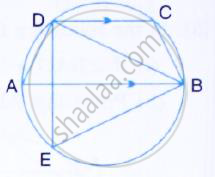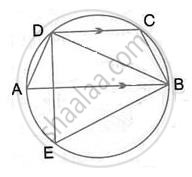Share

# Abcd is a Cyclic Quadrilateral in Which Ab is Parallel to Dc and Ab is a Diameter of the Circle. Given ∠Bed = 65°; Calculate: (I) ∠Dab, (Ii) ∠Bdc - Mathematics

Course

#### Question

ABCD is a cyclic quadrilateral in which AB is parallel to DC and AB is a diameter of the circle. Given ∠BED = 65°; Calculate:
(i) ∠DAB, (ii) ∠BDC#### Solution(i) ∠DAB = ∠BED = 65°
(Angle subtended by the same chord on the circle are equal)

(Angle in a semicircle is a right angle)
∴ ∠ABD = 90° - ∠DAB = 90° - 65° = 25°
AB || DC
∴ ∠BDC = ∠ABD = 25°  (Alternate angles)

Is there an error in this question or solution?